Next: Perturbed Lunar Motion Up: Lunar Motion Previous: Lunar Equations of Motion

Unperturbed Lunar Motion

Let us, first of all, neglect the perturbing influence of the Sun on the Moon's orbit by setting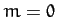in the lunar equations of motion (1151)-(1153). For the sake of simplicity, let us also neglect nonlinear effects in these equations by setting. In this case, the equations reduce to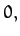(1157)(1158)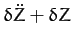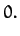(1159)

By inspection, appropriate solutions are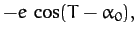(1160)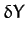(1161)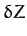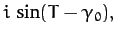(1162)

where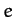,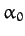,, andare arbitrary constants. Recalling that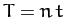, it follows from (1154)-(1156) that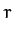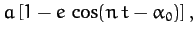(1163)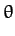(1164)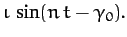(1165)

However, Equations (1163) and (1164) are simply first-order (in) approximations to the familiar Keplerian laws (see Chapter 5)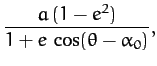(1166)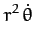(1167)

where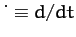. Of course, the above two laws describe a body which executes an elliptical orbit, confocal with the Earth, of major radius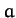, mean angular velocity, and eccentricity, such that the radius vector connecting the body to the Earth sweeps out equal areas in equal time intervals. We conclude, unsurprisingly, that the unperturbed lunar orbit is a Keplerian ellipse. Note that the lunar perigee lies at the fixed ecliptic longitude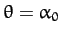. Equation (1165) is the first-order approximation to(1168)

This expression implies that the unperturbed lunar orbit is co-planar, but is inclined at an angle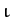to the ecliptic plane. Moreover, the ascending node lies at the fixed ecliptic longitude. Incidentally, the neglect of nonlinear terms in Equations (1157)-(1159) is only valid as long as,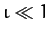: i.e., provided that the unperturbed lunar orbit is only slightly elliptical, and slightly inclined to the ecliptic plane. In fact, the observed values ofandare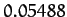and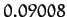radians, respectively, so this is indeed the case.Next: Perturbed Lunar Motion Up: Lunar Motion Previous: Lunar Equations of Motion
Richard Fitzpatrick 2011-03-31